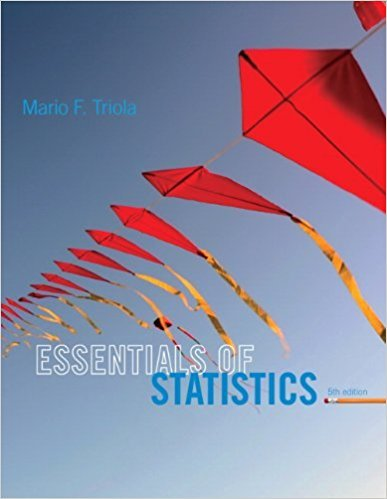×
×

# Scientific Notation. In Exercises 912, the givenISBN: 9780321924599 237

## Solution for problem 12 Chapter 1

Essentials of Statistics | 5th Edition

• Textbook Solutions
• 2901 Step-by-step solutions solved by professors and subject experts
• Get 24/7 help from StudySoup virtual teaching assistantsEssentials of Statistics | 5th Edition

4 5 1 351 Reviews
17
1
Problem 12

Scientific Notation. In Exercises 912, the given expressions are designed to yield re-sults expressed in a form of scientific notation. For example, the calculator-displayed re-sult of 1.23E5 can be expressed as 123,000, and the result of 4.56E-4 can be expressed as0.000456. Perform the indicated operation and express the result as an ordinary numberthat is not in scientific notation. 0.310

Step-by-Step Solution:
Step 1 of 3

STAT 110 – Notes for Week of 9/27/16  Chapter 13 Continued o Normal curve: a symmetric bell-shaped curve.  A normal curve is described by its mean and standard deviation.  The mean of a normal curve identifies the center of a distribution. The mean determines the location of the curve on a graph.  The standard deviation...

Step 2 of 3

Step 3 of 3

##### ISBN: 9780321924599

Unlock Textbook Solution